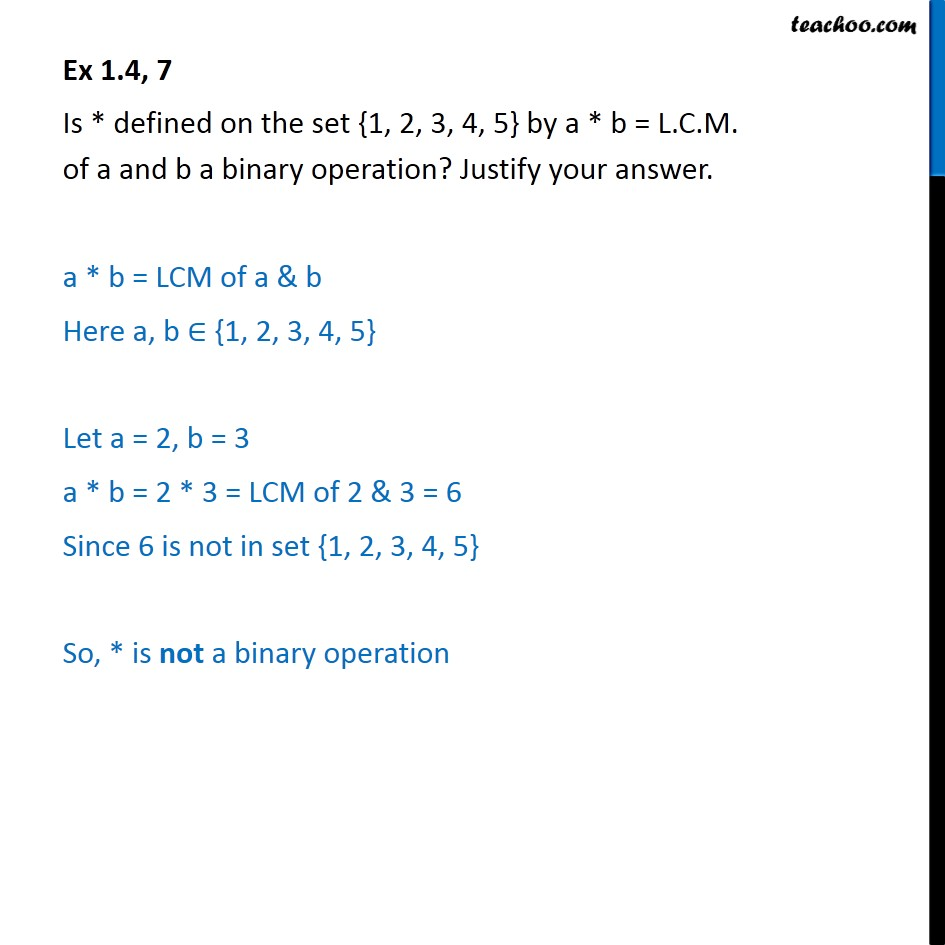Ex 1.4

Chapter 1 Class 12 Relation and Functions (Term 1)
Serial order wise### Transcript

Ex 1.4, 7 Is * defined on the set {1, 2, 3, 4, 5} by a * b = L.C.M. of a and b a binary operation? Justify your answer. a * b = LCM of a & b Here a, b ∈ {1, 2, 3, 4, 5} Let a = 2, b = 3 a * b = 2 * 3 = LCM of 2 & 3 = 6 Since 6 is not in set {1, 2, 3, 4, 5} So, * is not a binary operation